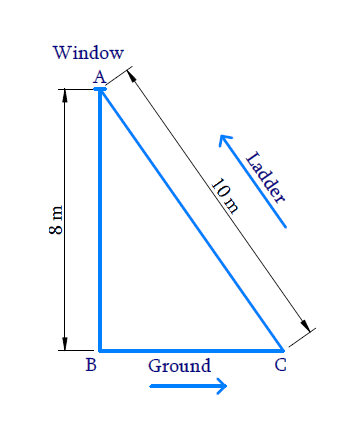# Ex.6.5 Q9 Triangles Solution - NCERT Maths Class 10

## Question

A ladder $$10$$$$\rm{}m$$ long reaches a window $$8$$$$\rm{}m$$ above the ground. Find the distance of the foot of the ladder from base of the wall.

DiagramVideo Solution
Triangles
Ex 6.5 | Question 9

## Text Solution

Reasoning:

In a right triangle, the square of the hypotenuse is equal to the sum of the squares of the other two sides.

Steps:

$$AB$$ is height of the windows from the ground $$\rm=8\,m$$

$$AC$$ is the length of the ladder $$\rm=10\,m$$

$$BC$$ is the foot of the ladder from the base of ground $$= ?$$

Since $$\Delta ABC$$ is right angled triangle $$(\angle ABC={{90}^{0}})$$

\begin{align} \Rightarrow B C^{2} &=A C^{2}-A B^{2} \\ [ \text { Pythagoras } &\text{ theorem } ] \\\\ B C^{2}&=10^{2}-8^{2} \\ B C^{2}&=100-64 \\ B C^{2} &=36 \\ B C &=6 \rm{}m \end{align}

The distance of the foot of the ladder from the base of the wall $$\rm=6\,m$$

Learn from the best math teachers and top your exams

• Live one on one classroom and doubt clearing
• Practice worksheets in and after class for conceptual clarity
• Personalized curriculum to keep up with school